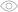# How Well Do You Know Variables and Constants in PHP?3041It's impossible to develop in any language without variables and constants, are you confident in this subject for the PHP language? Take the quiz and find out!

How are variables denoted in PHP?

variable_type \$variable_name;

variable_type variable_name;

\$variable_name;

What variable name is incorrect?

\$class_1

\$1class

\$_1class

Value 5 was set to the \$a variable, then the \$b=&\$a variable was declared, after that \$b was equated to 10. What are the values of \$a and \$b?

5, 10

5, 5

10, 10

Are \$b and \$c equal after this code execution?

\$c=7, \$b=1, since variables \$b and \$c aren't related by link and their values change independently of each other

Yes

No

How do you declare a constant correctly?

define (\$name, \$value, \$case_sensitivity);

const \$name = \$value;

\$name = value;

How to tell whether a constant was defined?

defined();

if(\$name) true;

echo \$name;

What system constant ...contains the name of the current file?

\$__FILENAME

\$FILE

\$__FILE__

How to enter text "1+3" into a variable?

\$x=1+3;

\$x="1+3";

string \$x="1+3";

What will be the result of code execution: echo \$_SERVER["PHP_SELF"];?

The server name will be entered

Nothing

The current file location will be entered

Is the \$__MYVAR__ name correct for a variable?

This is a correct declaration, but it’s better not to use it: PHP can register such a system constant once, that will disrupt the script functioning

Yes

Yes, but undesirable

No

It this link equating correctly: \$bar = &(24 * 7); ?

A link cannot direct an unnamed expression.

Yes

No

What will appear on the screen after code execution?

hello world world

hello world hello world

There will be an error

In what case will the \$_REQUEST['username']; variable have a value?

If the \$username variable has a value

If there’s a SWL request named username

If the username field was filled in a form

You know the subject at a basic level

Probably, you've only started to learn PHP and are in the dark about all the nuances of variable usage. Make sure to learn the theory and solve problems, and you'll definitely succeed! Share the quiz with friends and check their knowledge :)

You know the subject at middle level

You can use variables and constants correctly, however, more complex problems can raise difficulties. Train on problems, flip through a self-teaching textbook and you'll definitely succeed! Share the quiz with friends and check their knowledge :)

You know the subject in perfection

You mastered the subject and can use variables with no problems in any cases. Congrats! You can take on more complex subjects :) Share the quiz with friends and check their knowledge :)

How are variables denoted in PHP?
1 / 13
What variable name is incorrect?
2 / 13
Value 5 was set to the \$a variable, then the \$b=&amp;\$a variable was declared, after that \$b was equated to 10. What are the values of \$a and \$b?
3 / 13
Are \$b and \$c equal after this code execution?
4 / 13How do you declare a constant correctly?
5 / 13
How to tell whether a constant was defined?
6 / 13
What system constant ...contains the name of the current file?
7 / 13
How to enter text "1+3" into a variable?
8 / 13
What will be the result of code execution: echo \$_SERVER["PHP_SELF"];?
9 / 13
Is the \$__MYVAR__ name correct for a variable?
10 / 13
It this link equating correctly: \$bar = &amp;(24 * 7); ?
11 / 13
What will appear on the screen after code execution?
12 / 13In what case will the \$_REQUEST['username']; variable have a value?
13 / 13

### Calculating results...

Useful and short ads help us create new content every day.